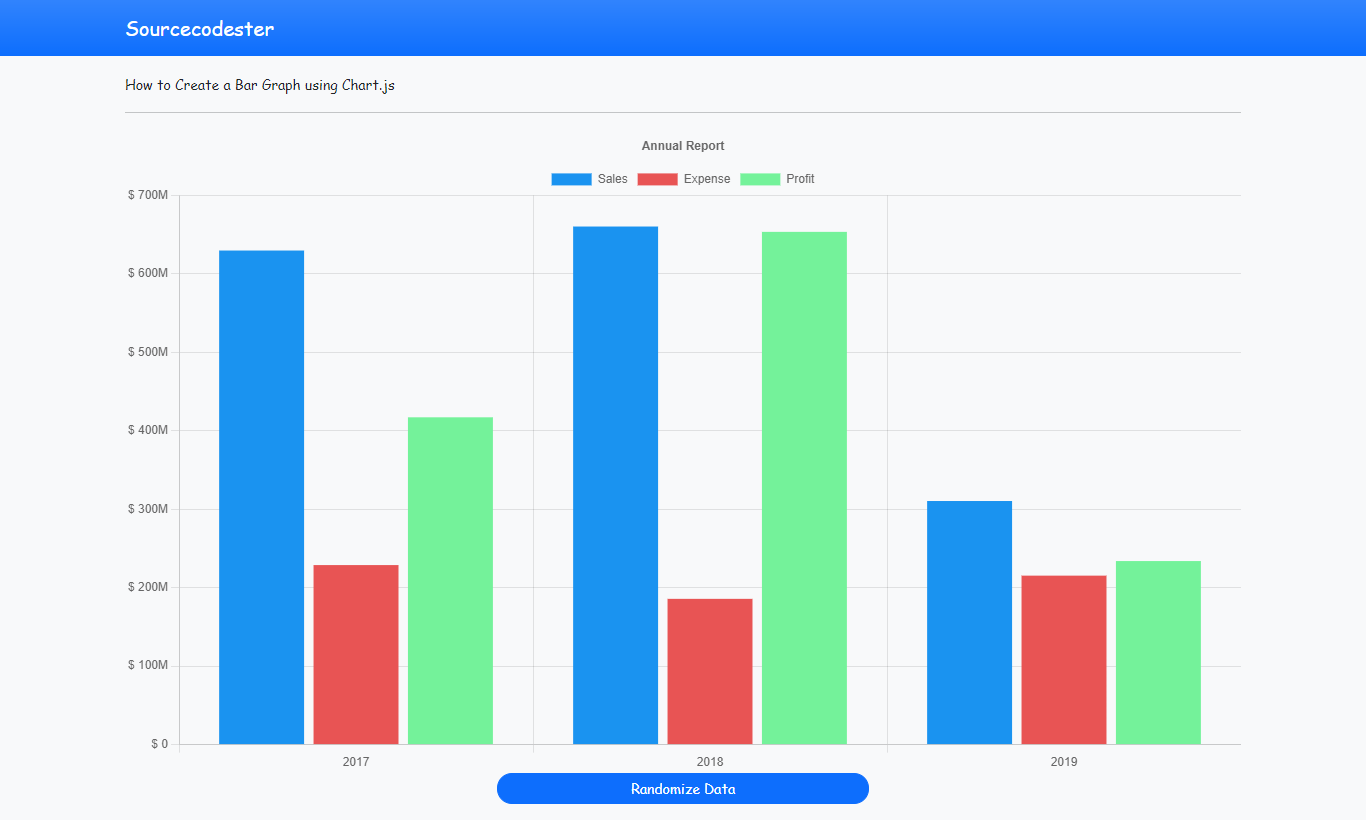# Creating a Simple Bar Graph using Chart.js Library Tutorial

Body

In this tutorial, we will tackle about how to create a Bar Graph using Chart.js. Chart.js is an open-source javascript library that focuses in generating a graphical canvases such as Line , Bar, and Pie Graphs. Here, I will be providin a source code that demonstrates how to generate a simple Bar Graph using the said javascript library.

The Bar Graph that I will show is a simple Annual Report within 3 years that has 3 types of data set each year which are the total Sales, Expenses, and Profit. I will be using Bootstrap page user-interface/design.

### Getting Started

In this tutorial source code, I usedBootstrap v5 for the design of application, jQuery Library, and the most important is Chart.js Library.

### Creating the Interface

First, I created an HTML scripts which contains the codes of the application interface. I save this file as index.html.

The code contains a simple top navigation bar, canvas tag where the graph will be shown, and a button for generate a new random data of the graph.

`<!DOCTYPE html><html lang="en"> <head>    <meta charset="UTF-8">    <meta http-equiv="X-UA-Compatible" content="IE=edge">    <meta name="viewport" content="width=device-width, initial-scale=1.0">    <title>Chart.js</title>    <link rel="stylesheet" href="./css/bootstrap.min.css">    <script src="./js/jquery-3.6.0.min.js"></script>    <script src="./js/bootstrap.min.js"></script>    <script src="./js/chart.min.js"></script>    <script src="./js/script.js"></script>    <style>         :root {            --bs-success-rgb: 71, 222, 152 !important;        }         html,        body {            height: 100%;            width: 100%;            font-family: Apple Chancery, cursive;        }    </style></head> <body class="bg-light">    <nav class="navbar navbar-expand-lg navbar-dark bg-primary bg-gradient" id="topNavBar">        <div class="container">            <a class="navbar-brand" href="https://sourcecodester.com">            Sourcecodester            </a>        </div>    </nav>    <div class="container py-3" id="page-container">        <small>How to Create a Bar Graph using Chart.js</small>        <hr>         <canvas id="chartCanvas" class="w-100" style="height: 60vh"></canvas>        <center><button class="btn btn-sm btn-primary rounded-pill col-md-4" type="button" onclick="regenerate()">Randomize Data</button></center>    </div> </body> </html>`

### Creating the Main Function

The following script is main script for the application which contains the Bar Charts Initilization, generating Randow Data values, and the function of Generating new Random Value of the Graph. I save this file as script.js.

`var ctx,    myChart;// Simple Function for generating a Ranfom valuefunction randomNumber() {    return Math.random() * (String(999999999).substring(Math.floor(Math.random * 9) + 3))} // Function for Generate a New Ranom Data of the Graphfunction regenerate() {    // looping the number of chart's data sets    Object.keys(myChart.data.datasets).map(k => {        var set = myChart.data.datasets[k];        // Updating the data values        set.data = [randomNumber(), randomNumber(), randomNumber()]    })     // Triggers Chart to display the updated Data    myChart.update()}\$(function() {    // Canvas Element where to display the Bar Graph    ctx = \$('#chartCanvas');    // Initializing the bar graph data and options    myChart = new Chart(ctx, {        type: 'bar',        data: {            labels: ['2017', '2018', '2019'],            datasets: [{                label: 'Sales',                data: [randomNumber(), randomNumber(), randomNumber()],                backgroundColor: "#1a93f0"            }, {                label: 'Expense',                data: [randomNumber(), randomNumber(), randomNumber()],                backgroundColor: "#e85454"            }, {                label: 'Profit',                data: [randomNumber(), randomNumber(), randomNumber()],                backgroundColor: "#74f29a"            }]        },        options: {            scales: {                y: {                    beginAtZero: true,                    ticks: {                        // Customizing Y Axes Label                        callback: function(value) {                            parseFloat(value)                            if (value >= 100000 && value < 1000000) {                                value = String(value).substring(0, 3) + "K"                            } else if (value >= 1000000 && value < 1000000000) {                                if (String(value).length == 7)                                    value = String(value).substring(0, 1) + "M"                                if (String(value).length == 8)                                    value = String(value).substring(0, 2) + "M"                                if (String(value).length == 9)                                    value = String(value).substring(0, 3) + "M"                            } else if (value >= 1000000000 && value <= 1000000000000) {                                if (String(value).length == 10)                                    value = String(value).substring(0, 1) + "B"                                if (String(value).length == 11)                                    value = String(value).substring(0, 2) + "B"                                if (String(value).length == 12)                                    value = String(value).substring(0, 3) + "B"                            } else {                                value = (value).toLocaleString('en-US')                            }                             return "\$ " + value;                        }                    }                }            },            plugins: {                legend: {                    display: true                },                title: {                    display: true,                    text: 'Annual Report'                }            }        }    });})`

### Result### DEMO VIDEO

That's the end of this tutorial. I hope this tutorial will help you and your future web application projects.

Explore more on this website for more Tutorials and Free Source Codes.